5G时代下一个聚合的编程学习网

# Excel & SQL | 日期和时间函数 | 05

## 获取当前时刻的数据

### 获取当前时刻的日期和时间

``````select now();
``````### 获取当前时刻的日期

now()函数获取的是当前时刻的日期和时间，有时候，我们可能只需要获取当前时刻的日期，并不需要获取当前时刻的时间，这个时候，在SQL中将now()函数换成curdate()函数，可以获取当前时刻的日期。

``````select curdate();
``````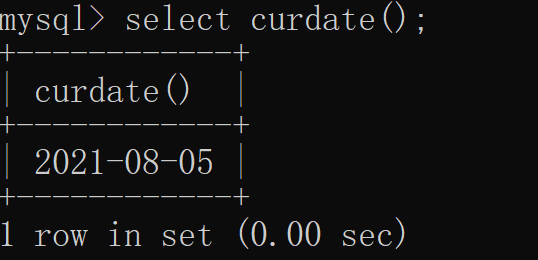curdate()函数用于直接获取当前时刻的日期，我们也可以先通过now()函数获取当前时刻的日期和时间，然后通过date()函数将日期和时间转化为日期，具体实现代码如下︰

``````select date(now());
``````

``````select year(now());
select month(now());
select day(now());
``````

### 获取当前时刻的时间

``````select curtime();
select time(now());
select hour(now()),minute(now()),second(now()); -- 分别获取时分秒
``````

### 获取当前时刻所属的周数

``````select weekforyear(now()); -- 查看这是今年的第几周
select dayofweek(now()); -- 注意：星期天是第一天
``````

### 获取当前时刻所属的季度

``````select quarter(now());
``````

## 日期和时间格式转换

``````date_format(datetime,format)
``````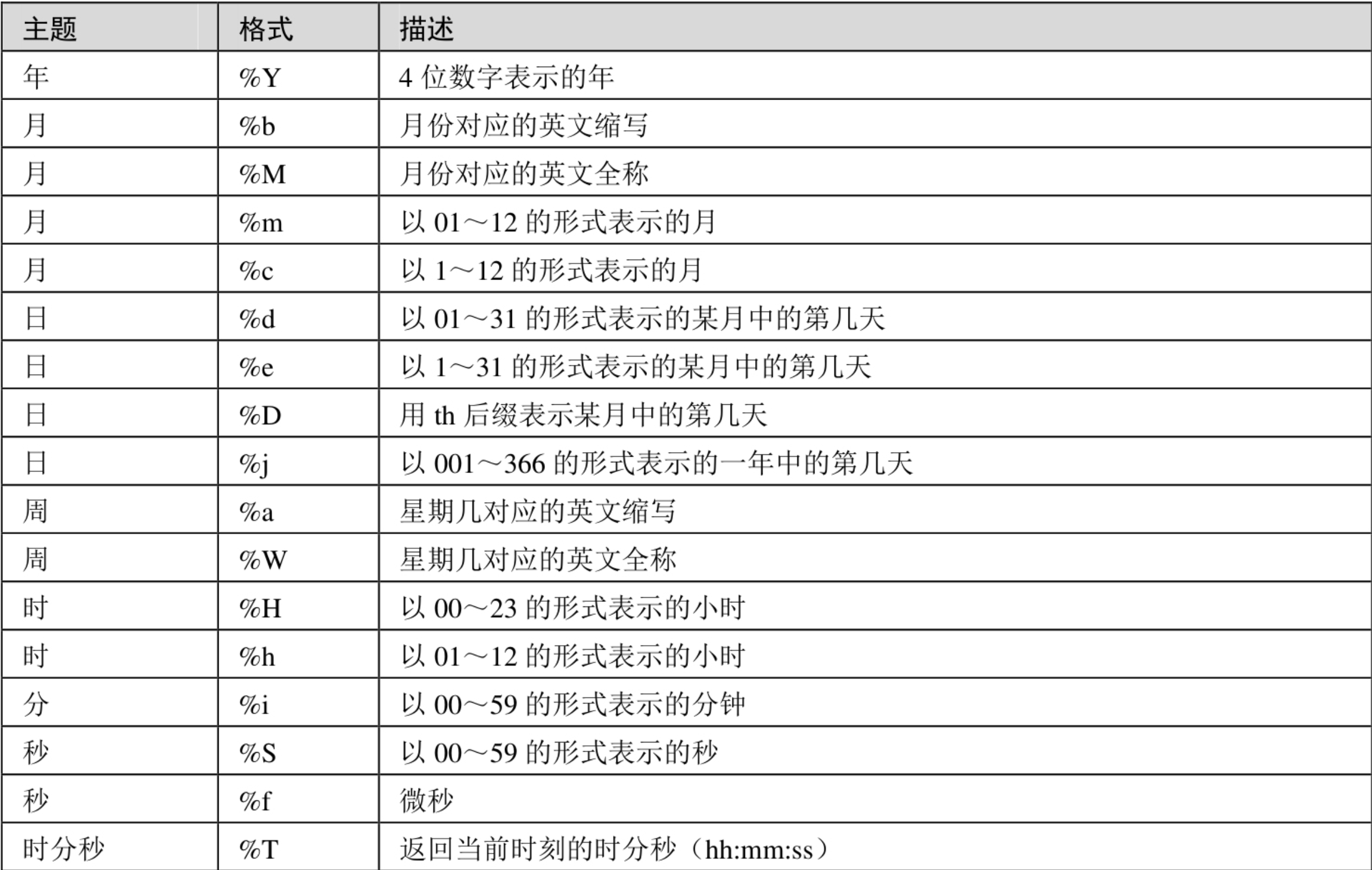``````select date_format(now(),"%Y-%m-%d %H:%i:%S");
``````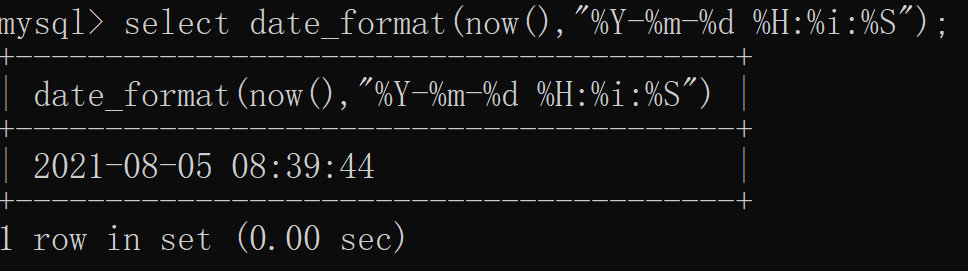``````extract(unit from datetime)
``````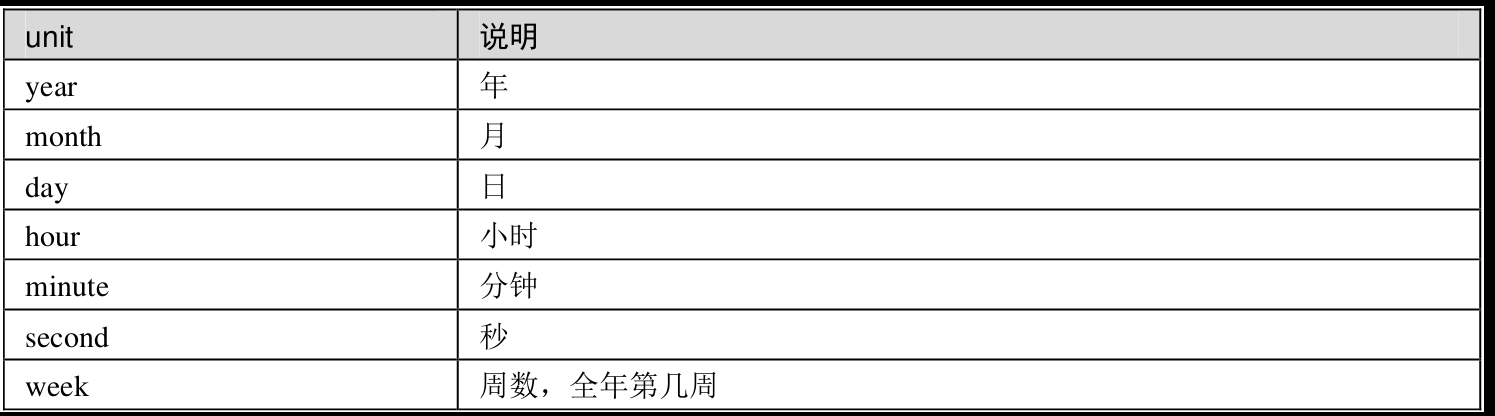``````select
extract(year from "2019-12-25 22:47:37") as col1,
extract(month from "2019-12-25 22:47:37") as col2,
extract(day from "2019-12-25 22:47:37") as col3;
``````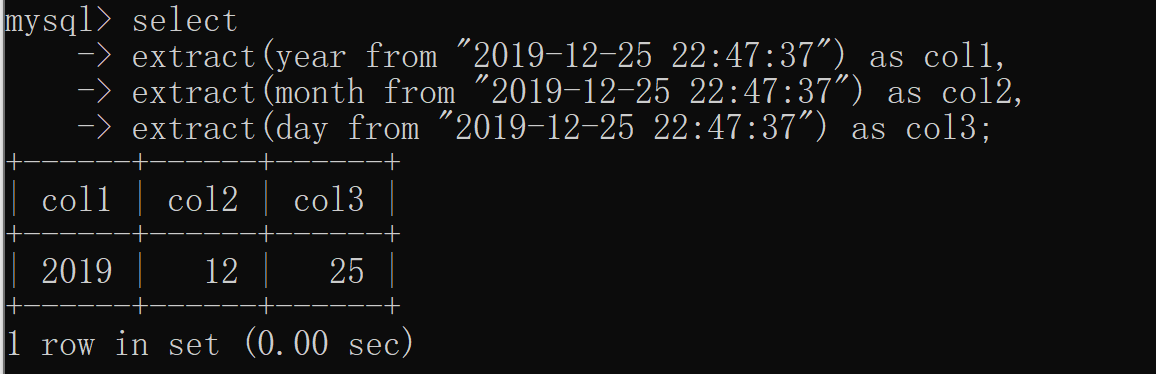## 日期和时间运算

### 向后偏移时间和日期

``````data_add(date,interval num unit)
``````

``````-- year,month,day,hour,minute,second
select date(now()) as col,date_add(date(now()),interval 7 year) as col2;
``````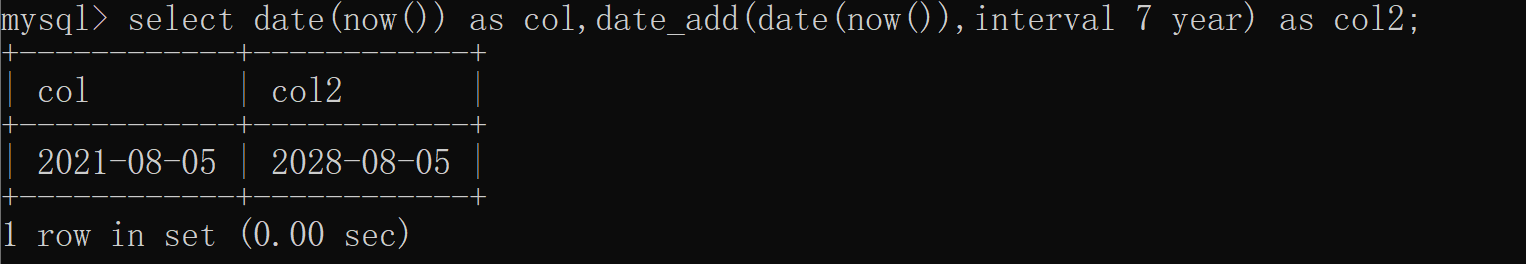### 向前偏移时间和日期

``````data_sub(date,interval num unit)
``````
``````-- year,month,day,hour,minute,second
select date(now()) as col,date_sub(date(now()),interval 7 year) as col2;
``````

### 两个日期做差

``````datediff(end_date,start_date)
``````

``````select datediff(date_add(now(),interval 8 day),date(now()));
``````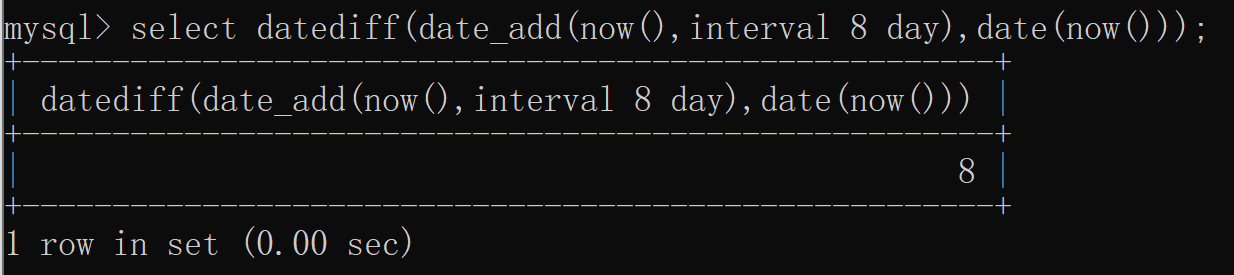### 两个日期之间的比较

``````select
"2019-01-01" > "2019-01-02" as col1,
"2019-01-01" < "2019-01-O2" as col2,
"2019-01-01" = "2019-01-02" as col3,
"2019-01-01" != "2019-01-02" as col4;
``````

## 小结

``````获取当前时刻的数据
获取当前日期和时间 select now;
获取当前日期 select curdate(); select date(now()); select year(now()),month(now()),day(now());
获取当前时间 select curtime(); select time(now());select hour(now()),minute(now()),second(now());
获取当前所属周数 weekofyear(now()) dayofweek(now())
获取当前所属季度 quarter(now());

格式转换 date_format(时间,格式) select date_format(now(),"%Y-%m-%d %H:%i:%S");
提取时间 extract(unit from 时间)
select
extract(year from "2019-12-25 22:47:37") as col1,
extract(month from "2019-12-25 22:47:37") as col2,
extract(day from "2019-12-25 22:47:37") as col3;

向后偏移时间和日期 date_sub(时间,interval num unit)
日期做差 datediff(end_time,start_time)
日期比较 两个日期之间的比较和两个数字之间的比较是一样的

``````